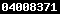### LATTICE ENERGY DETERMINATION AND MOLECULAR SIMULATION OF PEROVSKITE OXIDEShttps://doi.org/10.22146/ijc.21594

Hellna Tehubijuluw(1), Ismunandar Ismunandar(2*)

(1) Department of Chemistry, Faculty of Mathematics and Natural Science, State University of Pattimura, Ambon, Jl. Ir. M. Putuhena, Poka, Ambon 97123
(2) Department of Chemistry, Institute Technology of Bandung, Jl. Ganesha No. 10 Bandung 40132
(*) Corresponding Author

#### Abstract

Determination of lattice energy, U, is an important work for ionic compounds, due to it is the driving force in the compounds formation. The objective this work are twofold, firstly to determine lattice energy of perovskite oxide using an approximate equation and compare lattice energy from the resulted simple equation and molecular simulation results. The perovskite oxides used on this study were rare-earth orthoferite (LnFeO3) and aluminate (LnAlO3). These ionic terner compounds adopt space group Pbnm or R-3c. Lattice energy of perovskite oxides were calculated using Glasser, (G), Glasser-Jenkins, (GJ), and Yoder-Flora, (YF) equations. Plots of Born-Haber lattice energies (U(BHC)) vs. U(G), U(GJ) and U(YF) resulted in R2 = 0.8872, 0.5919, and 0.9982. Thus the obtained best fit was U(BHC) vs. U(YF). The simple equation describing the best plot wasUperovskite oxide =[1,0579U(YF) - 835,06] kJ/mol. Molecular simulation method has also been carried out to determine lattice energy and the obtained  results were compared with those obtained from simple lattice energy equation, good agreement were obtained in these two oxides series. The obtained structural parameters also agreed well with those obtained from X-ray diffraction studies.

Keywords: lattice energy, perovskite oxides, lattice energy equation, and molecular simulation

#### Full Text:

Full Text PdfDOI: https://doi.org/10.22146/ijc.21594

#### Article MetricsAbstract views : 492 |views : 1306

Copyright (c) 2010 Indonesian Journal of ChemistryView The Statistics of Indones. J. Chem.